library(MASS)
library(randomForest)
library(caret)

# R Packages

In this document, we will compare Random Forests and a similar method called Extremely Randomized Trees which can be found in the R package extraTrees. The extraTrees package uses Java in the background and sometimes has memory issues. The command below modifies the Java back-end to be given more memory by default. (By default the Java Virtual Machine is allocated 512 MB, which we change to 4 GB.) This must be done before loading the extraTrees package.

options(java.parameters = "-Xmx4g")
library(extraTrees)

Details on the R package can be found in its vignette.

We will also discuss ranger an alternative package for fitting a random forest, as well as xgboost, an alternative boosting package.

# Extremely Randomized Trees

Extremely Randomized Trees (ERT) are very similar to Random Forests. (RF) There are essentially two main differences:

• ERT do not resample observations when building a tree. (They do not perform bagging.)
• ERT do not use the “best split.”
• Like a RF, ERT select a random subset of predictors for each split. (A tuning parameter: mtry)
• Instead of the “best split” for the predictors, ERT makes a small number of randomly chosen splits-points for each of the selected predictors. In the original method, this value was 1. (A tuning parameter: numRandomCuts)
• ERT then selects the “best split” from this small number of choices.

The resulting “forest” contains trees that are more variable, but less correlated than the trees in a Random Forest. Details of the method can be found in the original paper.

As most papers do, the claim is that Extremely Randomized Trees are better than Random Forests. In practice, you will find this is certainly true sometimes, but not always. Remember, there is no free lunch.

ERT can be used for both classification and regression, much like a RF. We will evaluate a regression example in this document.

# Regression Example

We consider the regression case, using the Boston data from the MASS package. We will use RMSE as our metric, so we write a function which will help us along the way:

rmse = function(actual, predicted) {
sqrt(mean((actual - predicted) ^ 2))
}

As always, we test-train split the data. Half for training, half for testing in this case.

set.seed(42)
boston_idx = sample(1:nrow(Boston), nrow(Boston) / 2)
boston_trn = Boston[boston_idx,]
boston_tst = Boston[-boston_idx,]

Notice that this dataset contains 13 predictor variables.

## Random Forest

We first train a Random Forest model. For this example, we will use cross-validation to select a value of mtry, the tuning parameter for RF. Two reasons for this:

• OOB error calculations are not implemented for the extraTrees package. So we’ll use CV for both to keep the comparison as similar as possible.
• Using CV allows us to create a nice plot of the results.

We setup both our cross-validation (5 fold) and a grid of mtry values. (Here, trying all possible values.)

cv_5 = trainControl(method = "cv", number = 5)
rf_grid =  expand.grid(mtry = 1:13)

We then train the model.

set.seed(42)
rf_fit = train(medv ~ ., data = boston_trn,
method = "rf",
trControl = cv_5,
tuneGrid = rf_grid)

We suppress the bulk of the output and only view the selected tuning parameters and a plot of our results.

#rf_fit
rf_fit$bestTune ## mtry ## 7 7 plot(rf_fit)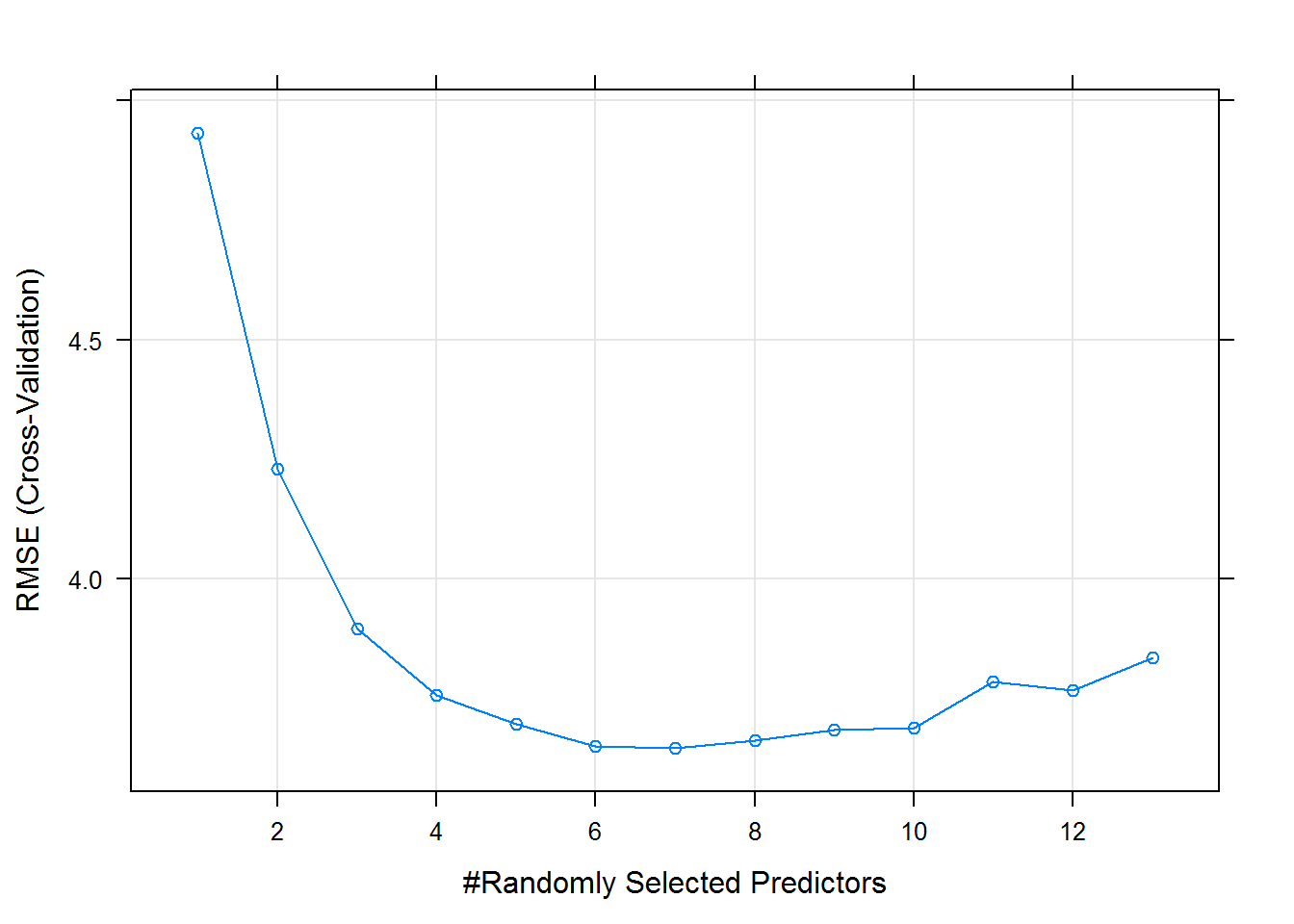rmse(predict(rf_fit, boston_tst), boston_tst$medv)
##  4.122169

We find the resulting test RMSE for our chosen RF model with mtry = 7 to be 4.1221691.

## Extremely Randomized Trees

We now try an Extremely Randomized Trees model. The ERT model has two parameters:

• mtry which works in the same way as RF.
• numRandomCuts which determines the number of randomly chosen splits for each of the mtry predictors selected for each split. Lower values make trees more random.

When specifying the grid of values, we only use selected values of mtry and only “small” values of numRandomCuts to keep computation time somewhat reasonable. (Remember we’re cross-validating which takes more time than using OOB samples.) Usually numRandomCuts is probably kept smaller than these values, say 1:5 but these values were chosen for the plot below. A value of 1 is the value for the originally specified ERT method.

et_grid =  expand.grid(mtry = 4:7, numRandomCuts = 1:10)

We train the model using caret with method = "extraTrees" which uses the extraTrees package. When training the model, we add one extra argument, numThreads = 4 which tells R to use 4 cores in the Java Virtual Machine. (Which will speed up computation.)

set.seed(42)
et_fit = train(medv ~ ., data = boston_trn,
method = "extraTrees",
trControl = cv_5,
tuneGrid = et_grid,
numThreads = 4)

Again, we suppress the bulk of the output and only view the selected tuning parameters and a plot of our results.

#et_fit
et_fit$bestTune ## mtry numRandomCuts ## 33 7 3 plot(et_fit)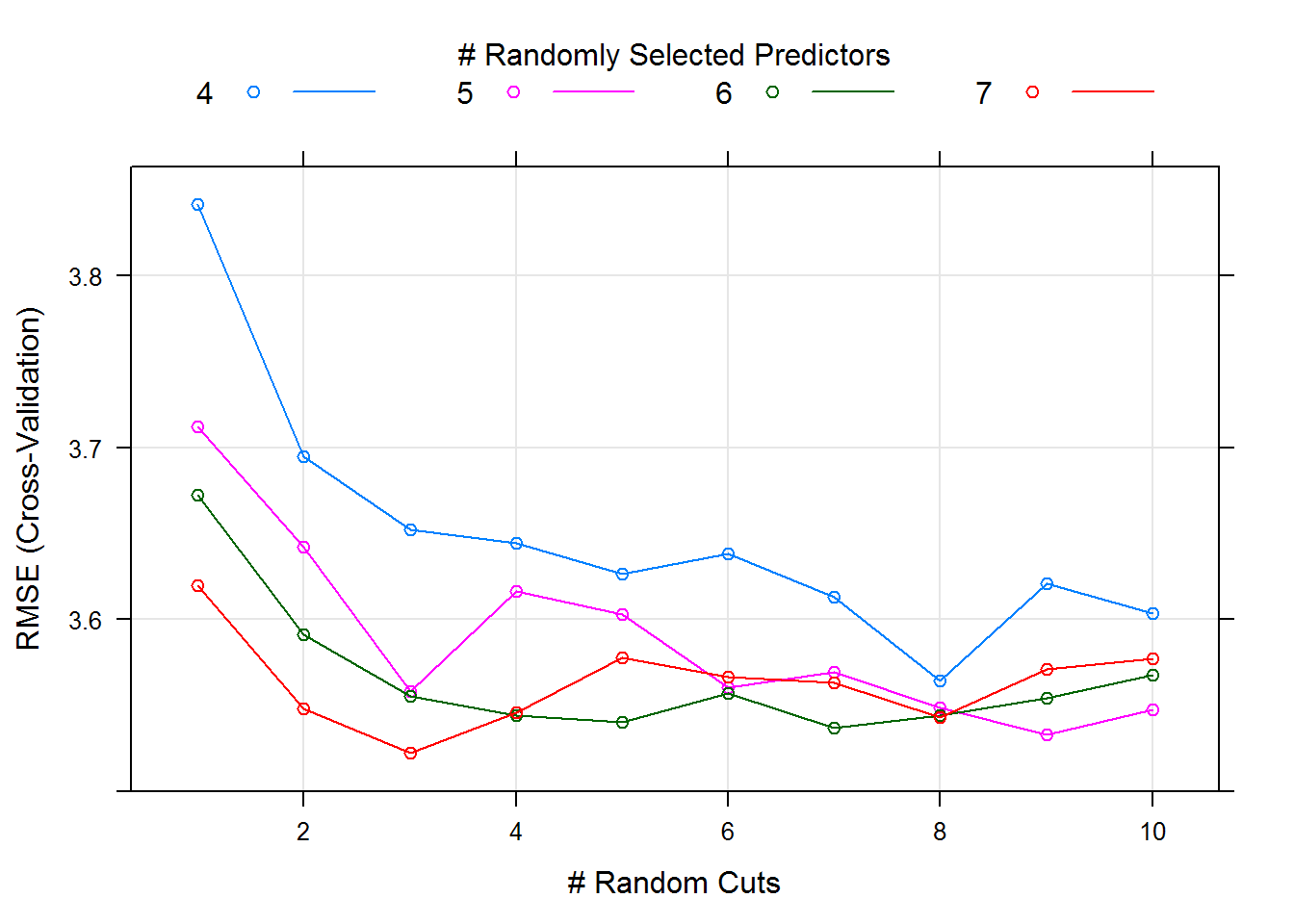rmse(predict(et_fit, boston_tst), boston_tst$medv)
##  3.882574

We find the resulting test RMSE for our chosen ERT model to be 3.882574. So, for this example, Extremely Randomized Trees win over Random Forests, but remember this won’t always be the case.

# ranger

The ranger package simply re-implements the random forest method. It has a number of speed advantages, including the ability to grow trees in parallel.

library(ranger)
set.seed(42)
system.time({ranger_fit = train(medv ~ ., data = boston_trn,
method = "ranger",
trControl = cv_5,
tuneGrid = rf_grid)})
##    user  system elapsed
##    9.84    0.50   10.17
set.seed(42)
system.time({ranger_fit = train(medv ~ ., data = boston_trn,
method = "ranger",
trControl = cv_5,
tuneGrid = rf_grid)})
##    user  system elapsed
##   10.22    0.34    3.81
#ranger_fit
ranger_fit$bestTune ## mtry ## 7 7 plot(ranger_fit)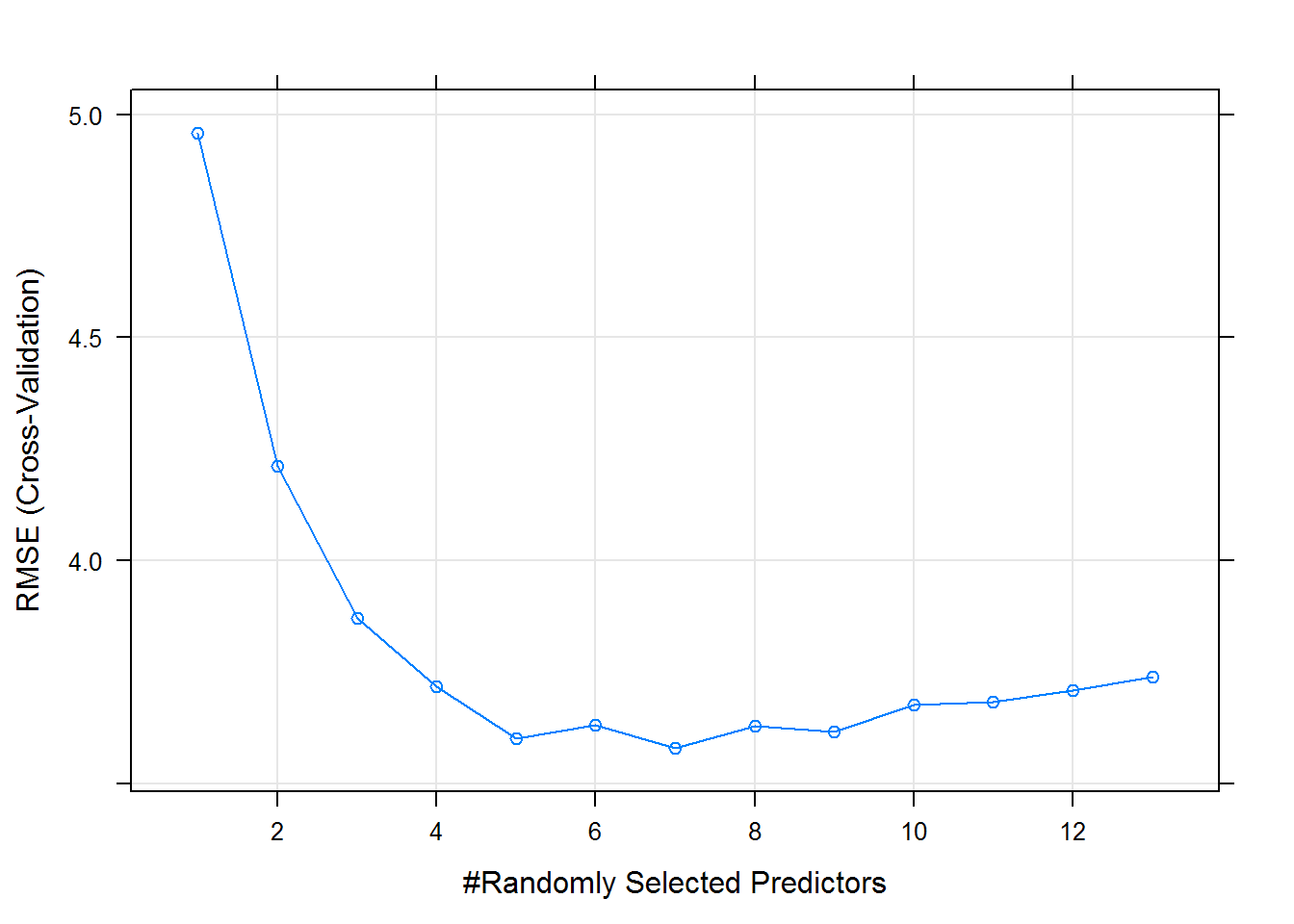rmse(predict(ranger_fit, boston_tst), boston_tst$medv)
##  4.253865

Notice due to the differences in the implementation, the results are not the same as the original random forest. In general the results should be similar.

# xgboost

The xgboost package implements eXtreme Gradient Boosting, which is similar to the methods found in gbm. Tuned well, often xgboost can obtain excellent results, often winning Kaggle competitions. (In this example it beats gbm, but not the random forest based methods.)

library(gbm)
library(xgboost)
set.seed(42)
gbm_fit = train(medv ~ ., data = boston_trn,
method = "gbm",
trControl = cv_5,
verbose = FALSE,
tuneLength = 10)
#gbm_fit
gbm_fit$bestTune ## n.trees interaction.depth shrinkage n.minobsinnode ## 71 50 8 0.1 10 plot(gbm_fit)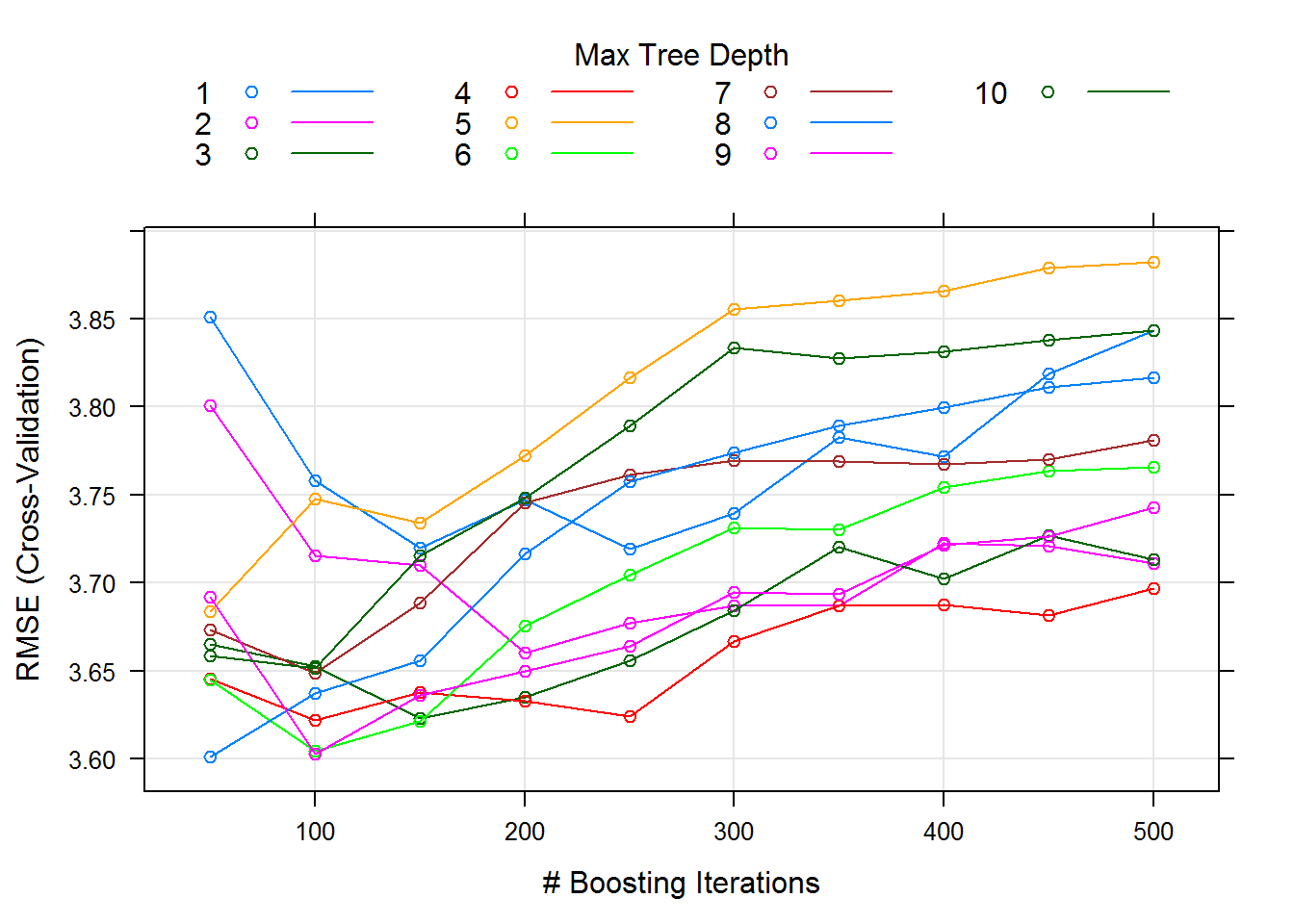rmse(predict(gbm_fit, boston_tst), boston_tst$medv)
##  4.488456
set.seed(42)
xgb_fit = train(medv ~ ., data = boston_trn,
method = "xgbTree",
trControl = cv_5,
verbose = FALSE,
tuneLength = 10)
#xgb_fit
xgb_fit$bestTune ## nrounds max_depth eta gamma colsample_bytree min_child_weight ## 373 150 9 0.4 0 0.8 1 plot(xgb_fit)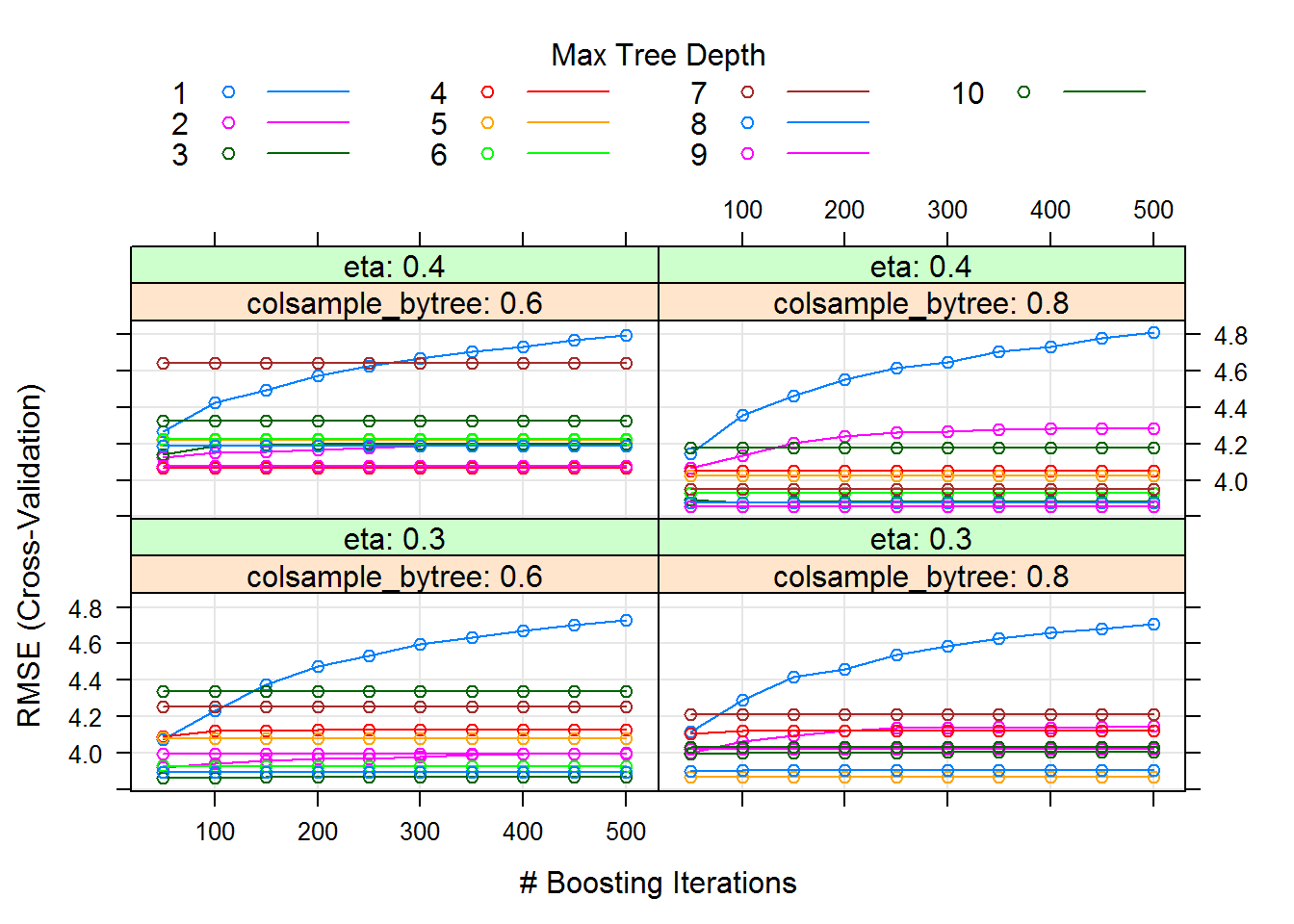rmse(predict(xgb_fit, boston_tst), boston_tst$medv)
##  4.003484